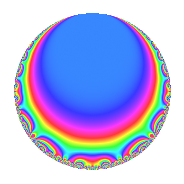# Properties

 Label 4005.2.a.fLevel 4005 Weight 2 Character orbit 4005.a Self dual Yes Analytic conductor 31.980 Analytic rank 1 Dimension 2 CM No Inner twists 1

# Related objects

## Newspace parameters

 Level: $$N$$ = $$4005 = 3^{2} \cdot 5 \cdot 89$$ Weight: $$k$$ = $$2$$ Character orbit: $$[\chi]$$ = 4005.a (trivial)

## Newform invariants

 Self dual: Yes Analytic conductor: $$31.9800860095$$ Analytic rank: $$1$$ Dimension: $$2$$ Coefficient field: $$\Q(\sqrt{3})$$ Coefficient ring: $$\Z[a_1, a_2]$$ Coefficient ring index: $$1$$ Fricke sign: $$1$$ Sato-Tate group: $\mathrm{SU}(2)$

## $q$-expansion

Coefficients of the $$q$$-expansion are expressed in terms of $$\beta = \sqrt{3}$$. We also show the integral $$q$$-expansion of the trace form.

 $$f(q)$$ $$=$$ $$q$$ $$+ \beta q^{2}$$ $$+ q^{4}$$ $$- q^{5}$$ $$+ ( -1 + \beta ) q^{7}$$ $$-\beta q^{8}$$ $$+O(q^{10})$$ $$q$$ $$+ \beta q^{2}$$ $$+ q^{4}$$ $$- q^{5}$$ $$+ ( -1 + \beta ) q^{7}$$ $$-\beta q^{8}$$ $$-\beta q^{10}$$ $$+ 2 q^{13}$$ $$+ ( 3 - \beta ) q^{14}$$ $$-5 q^{16}$$ $$+ ( -1 - \beta ) q^{19}$$ $$- q^{20}$$ $$+ ( -3 - \beta ) q^{23}$$ $$+ q^{25}$$ $$+ 2 \beta q^{26}$$ $$+ ( -1 + \beta ) q^{28}$$ $$-2 \beta q^{29}$$ $$+ ( -1 + 3 \beta ) q^{31}$$ $$-3 \beta q^{32}$$ $$+ ( 1 - \beta ) q^{35}$$ $$+ ( -4 + 2 \beta ) q^{37}$$ $$+ ( -3 - \beta ) q^{38}$$ $$+ \beta q^{40}$$ $$-2 \beta q^{41}$$ $$+ ( 11 + \beta ) q^{43}$$ $$+ ( -3 - 3 \beta ) q^{46}$$ $$+ ( -6 - 4 \beta ) q^{47}$$ $$+ ( -3 - 2 \beta ) q^{49}$$ $$+ \beta q^{50}$$ $$+ 2 q^{52}$$ $$-4 \beta q^{53}$$ $$+ ( -3 + \beta ) q^{56}$$ $$-6 q^{58}$$ $$+ ( -9 + 3 \beta ) q^{59}$$ $$+ 2 q^{61}$$ $$+ ( 9 - \beta ) q^{62}$$ $$+ q^{64}$$ $$-2 q^{65}$$ $$+ ( -4 - 6 \beta ) q^{67}$$ $$+ ( -3 + \beta ) q^{70}$$ $$+ ( -6 - 2 \beta ) q^{71}$$ $$+ ( 2 - 4 \beta ) q^{73}$$ $$+ ( 6 - 4 \beta ) q^{74}$$ $$+ ( -1 - \beta ) q^{76}$$ $$+ ( 2 - 6 \beta ) q^{79}$$ $$+ 5 q^{80}$$ $$-6 q^{82}$$ $$+ ( 3 - 3 \beta ) q^{83}$$ $$+ ( 3 + 11 \beta ) q^{86}$$ $$+ q^{89}$$ $$+ ( -2 + 2 \beta ) q^{91}$$ $$+ ( -3 - \beta ) q^{92}$$ $$+ ( -12 - 6 \beta ) q^{94}$$ $$+ ( 1 + \beta ) q^{95}$$ $$-4 q^{97}$$ $$+ ( -6 - 3 \beta ) q^{98}$$ $$+O(q^{100})$$ $$\operatorname{Tr}(f)(q)$$ $$=$$ $$2q$$ $$\mathstrut +\mathstrut 2q^{4}$$ $$\mathstrut -\mathstrut 2q^{5}$$ $$\mathstrut -\mathstrut 2q^{7}$$ $$\mathstrut +\mathstrut O(q^{10})$$ $$2q$$ $$\mathstrut +\mathstrut 2q^{4}$$ $$\mathstrut -\mathstrut 2q^{5}$$ $$\mathstrut -\mathstrut 2q^{7}$$ $$\mathstrut +\mathstrut 4q^{13}$$ $$\mathstrut +\mathstrut 6q^{14}$$ $$\mathstrut -\mathstrut 10q^{16}$$ $$\mathstrut -\mathstrut 2q^{19}$$ $$\mathstrut -\mathstrut 2q^{20}$$ $$\mathstrut -\mathstrut 6q^{23}$$ $$\mathstrut +\mathstrut 2q^{25}$$ $$\mathstrut -\mathstrut 2q^{28}$$ $$\mathstrut -\mathstrut 2q^{31}$$ $$\mathstrut +\mathstrut 2q^{35}$$ $$\mathstrut -\mathstrut 8q^{37}$$ $$\mathstrut -\mathstrut 6q^{38}$$ $$\mathstrut +\mathstrut 22q^{43}$$ $$\mathstrut -\mathstrut 6q^{46}$$ $$\mathstrut -\mathstrut 12q^{47}$$ $$\mathstrut -\mathstrut 6q^{49}$$ $$\mathstrut +\mathstrut 4q^{52}$$ $$\mathstrut -\mathstrut 6q^{56}$$ $$\mathstrut -\mathstrut 12q^{58}$$ $$\mathstrut -\mathstrut 18q^{59}$$ $$\mathstrut +\mathstrut 4q^{61}$$ $$\mathstrut +\mathstrut 18q^{62}$$ $$\mathstrut +\mathstrut 2q^{64}$$ $$\mathstrut -\mathstrut 4q^{65}$$ $$\mathstrut -\mathstrut 8q^{67}$$ $$\mathstrut -\mathstrut 6q^{70}$$ $$\mathstrut -\mathstrut 12q^{71}$$ $$\mathstrut +\mathstrut 4q^{73}$$ $$\mathstrut +\mathstrut 12q^{74}$$ $$\mathstrut -\mathstrut 2q^{76}$$ $$\mathstrut +\mathstrut 4q^{79}$$ $$\mathstrut +\mathstrut 10q^{80}$$ $$\mathstrut -\mathstrut 12q^{82}$$ $$\mathstrut +\mathstrut 6q^{83}$$ $$\mathstrut +\mathstrut 6q^{86}$$ $$\mathstrut +\mathstrut 2q^{89}$$ $$\mathstrut -\mathstrut 4q^{91}$$ $$\mathstrut -\mathstrut 6q^{92}$$ $$\mathstrut -\mathstrut 24q^{94}$$ $$\mathstrut +\mathstrut 2q^{95}$$ $$\mathstrut -\mathstrut 8q^{97}$$ $$\mathstrut -\mathstrut 12q^{98}$$ $$\mathstrut +\mathstrut O(q^{100})$$

## Embeddings

For each embedding $$\iota_m$$ of the coefficient field, the values $$\iota_m(a_n)$$ are shown below.

For more information on an embedded modular form you can click on its label.

Label $$\iota_m(\nu)$$ $$a_{2}$$ $$a_{3}$$ $$a_{4}$$ $$a_{5}$$ $$a_{6}$$ $$a_{7}$$ $$a_{8}$$ $$a_{9}$$ $$a_{10}$$
1.1
 −1.73205 1.73205
−1.73205 0 1.00000 −1.00000 0 −2.73205 1.73205 0 1.73205
1.2 1.73205 0 1.00000 −1.00000 0 0.732051 −1.73205 0 −1.73205
 $$n$$: e.g. 2-40 or 990-1000 Significant digits: Format: Complex embeddings Normalized embeddings Satake parameters Satake angles

## Inner twists

This newform does not admit any (nontrivial) inner twists.

## Atkin-Lehner signs

$$p$$ Sign
$$3$$ $$-1$$
$$5$$ $$1$$
$$89$$ $$-1$$

## Hecke kernels

This newform can be constructed as the intersection of the kernels of the following linear operators acting on $$S_{2}^{\mathrm{new}}(\Gamma_0(4005))$$:

 $$T_{2}^{2}$$ $$\mathstrut -\mathstrut 3$$ $$T_{7}^{2}$$ $$\mathstrut +\mathstrut 2 T_{7}$$ $$\mathstrut -\mathstrut 2$$ $$T_{11}$$